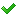•闪存
•博客
• 发言小组
• 投递新闻
• 提问博问
• 添加收藏
• 发布招聘
•文库

1

3

2

1

##opencv

cv::cvtColor(inputMat, greyMat, CV_BGR2GRAY);中第一个参数 格式怎么赋值啊，我有一个oc的字符串代表图片地址，怎么把这个字符串赋值到第一个参数中啊
1

1

4

1

1

##5Python ---opencv

import cv2import numpy as npimg1 = cv2. imread( 'star.jpg' , 0)img2 = cv2. imread( 'star2.jpg' , 0)r
1

1

1

##5python cv.imread()第二个参数

import cv2import numpyimport matplotlib.pyplot as plimg=cv2.imread("D:\\cat.jpg",0) pl.imshow(img,cm
2

6